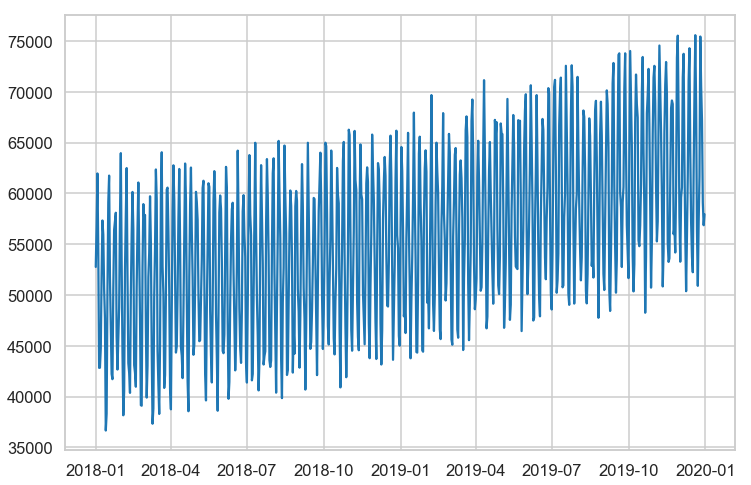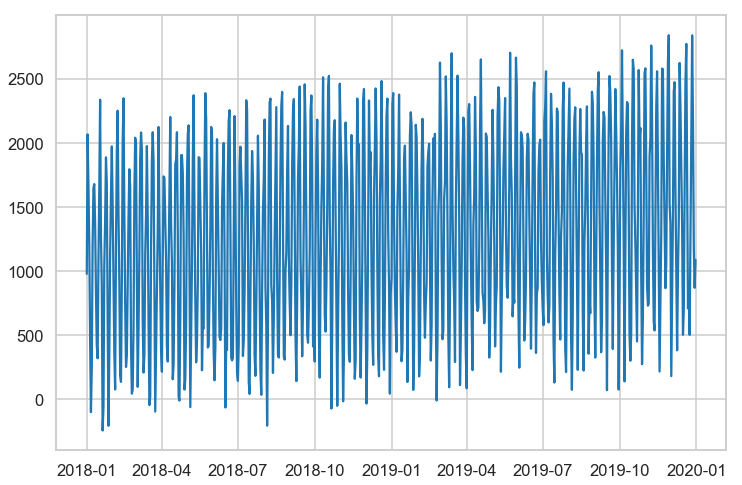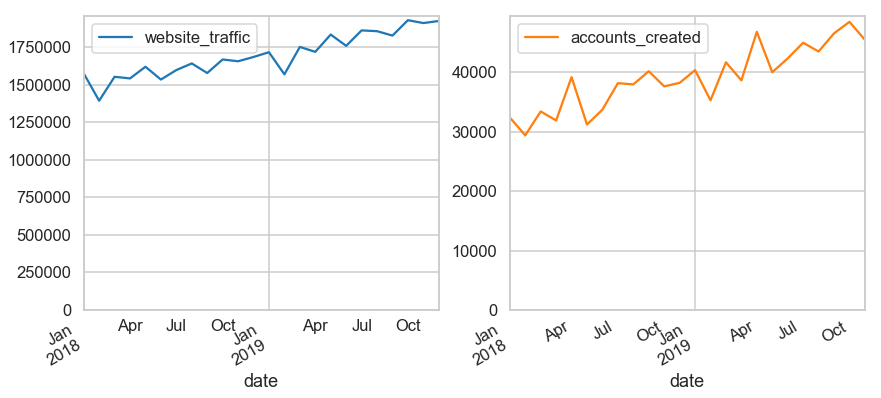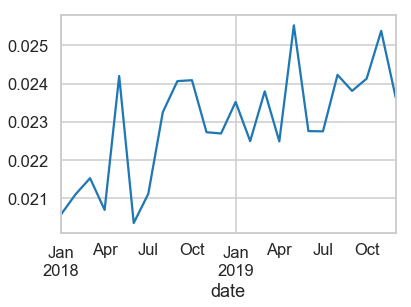Numerous times I've found myself in a situation where data is not yet in a datawarehouse or available in a visualization tool (such as Looker), but nonetheless charts were expected for scheduled reports. This is a gallery of some of the charts I've made. It's important to note that Chris Moffit's post on Effectively Using Matplotlib was instrumental to finally grokking how to interact with that charting library.

```%matplotlib inline
import numpy as np
from numpy.random import randn
import pandas as pd
import matplotlib.pyplot as plt
import seaborn as sns
sns.set_style('whitegrid')
sns.set_context("talk")

np.random.seed(12345)
```

## Create Some Fake Data

Most of the charts I deal with in Marketing Analytics / Business Operations have been based on timeseries data. In prepping for this post, I found simulating timeseries, especially the patterns I see frequently in marketing analytics, not as straightforward. Luckily a few different people on Twitter were helpful, especially this suggestion from Shahar Shani-Kadmiel:

```# set the time range
time = pd.date_range(
start = '2018-01-01',
end = '2019-12-31',
freq = 'D'
)

# leverage sine waves to create daily data with weekly patterns and a trend that shows growth over time
amp = 1000
freq = 52 / 365
data = amp * np.sin(freq * 2 * np.pi * np.arange(time.size))
noise = 2 * np.random.rand(time.size) - 1
data += 0.2 * data.ptp() * noise
trend = np.power(np.arange(time.size),1.25)
data += trend
data += 50000
data = np.around(data,decimals=0).astype(int)
```
```def generate_series(time,amp,freq,power,vertical_shift):
data = amp * np.sin(freq * 2 * np.pi * np.arange(time.size))
noise = 2 * np.random.rand(time.size) - 1
data += 0.2 * data.ptp() * noise
trend = np.power(np.arange(time.size),power)
data += trend
data += vertical_shift
data = np.around(data,decimals=0).astype(int)
return data
```
```date_range = pd.date_range(start = '2018-01-01', end = '2019-12-31',freq = 'D')
```
```web_traffic = generate_series(date_range,10000,(52 / 365),1.45,50000)

fig, ax = plt.subplots(figsize=(12,8))
ax.plot(date_range,web_traffic)
```
`[<matplotlib.lines.Line2D at 0x1c367906a0>]````acct_create = generate_series(date_range,1000,(52 / 365),0.95,1000)

fig, ax = plt.subplots(figsize=(12,8))
ax.plot(date_range,acct_create)
```
`[<matplotlib.lines.Line2D at 0x1c36d462b0>]````traffic = pd.DataFrame(web_traffic,index=time,columns=['website_traffic'])
traffic.index.name = 'date'
```
```accts = pd.DataFrame(acct_create,index=time,columns=['accounts_created'])
accts.index.name = 'date'
```
```df = pd.concat([traffic,accts],axis=1)
df = df.reset_index()
```
date website_traffic accounts_created
0 2018-01-01 52765 976
1 2018-01-02 56745 2065
2 2018-01-03 61969 1675
3 2018-01-04 54372 1212
4 2018-01-05 42823 474
```df.groupby(pd.Grouper(key='date',freq='MS')).sum().plot(subplots=True, layout=(1,2), figsize=(14, 6), sharey=False, ylim = 0);
``````df.groupby(pd.Grouper(key='date',freq='MS')).sum().assign(signup_rate = lambda x: x['accounts_created'] / x['website_traffic']).loc[:,'signup_rate'].plot()
```
`<matplotlib.axes._subplots.AxesSubplot at 0x1c37b0a358>`